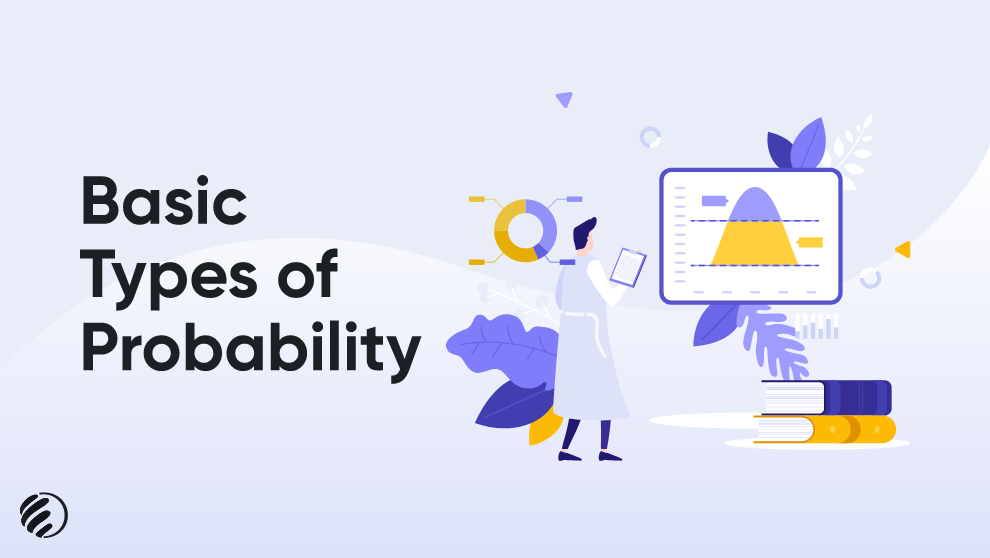# Basic Types of Probability500 Views10 Mins To ReadCategory: CalculatorsSeptember 2, 2022

Probability is a term that is used to analyze the chances of an event occurring. In simple words, you can take probability as a possibility. Will it rain today or not? Will you get a head once you flip the coin? These are some basic examples of probability in which both events can occur, and you can’t say anything for sure. Probability ranges from 0 to 1, where 0 means impossible and 1 means possible. Probability is widely used in various fields of life these days. For example, meteorologists use weather patterns to forecast the likelihood of rain. Probability is commonly used in epidemiology to comprehend the connections between exposures and the risk of health issues.

## Types of Probability

Probability is a broad term that has various types. An individual needs to know about the types of probability to get a better understanding of the topic. The primary types of probability are discussed below.

### 1. Theoretical Probability

This type of probability is mainly based on the reasoning behind the chances of occurring an event. For instance, you don’t have enough time to analyze the results of dice by throwing them hundreds of times; then, you can calculate probabilities on the grounds of some mathematical theory and rules.
When you toss a die, you will find that all sides have equal chances to land face-up. Thus, the possibility of rolling a 6 is 1/6 for a six-sided die.

### 2. Experimental Probability

The experimental probability completely lies in the observations of an experiment. This type of probability can be found by calculating the number of possible outcomes with respect to the total number of trials. For instance, if you toss a dice 15 times, and you get 6 for 7 times, then the experimental probability of getting 6 is 6/15, or 2/5.

### 3. Axiomatic Probability

A set of axioms (rules) are made in axiomatic probability, which is applied to all types. Kolmogorov designed these axioms’ rules; therefore, they are also known as Kolmogorov’s three axioms.
The axiomatic approach to probability enables an individual to discover an event’s chances of occurrence or non-occurrence. The axiomatic phenomenon explains that probability is any function (P) from events to the total numbers filling the three conditions (axioms).

## The three axioms of probability

• 0 ≤ P(E) ≤ 1 for every possible event E. You can simply say that 0 is the smallest number and 1 is the largest possible event.
• The definite event has probability 1. The definite events are the ones that will occur for sure. In the example of rolling dice, you can be sure that possible events will be from 1,2,3,4,5,6. The results you will get will be from these certain events.
• The possibility of the unification of mutually exclusive events is the total of the chances of the individual events.

For instance, if A is an event, “1 comes up on the die” and B is the event “an even number will come on dice,” then the combination of A and B is the event then the results you will get on the dice will be either 1 or even. Any manual process takes time, so if you want to verify single or multiple probabilities without wasting time, use an online probability calculator.

#### At the End

The possibility of occurrence or non-occurrence of some events is known as probability. This process is widely used in different fields of life. It is mostly used to find out the chances of an event occurring, so the required steps can be planned accordingly. Use an online probability calculator on searchenginereports.net to get error-free results in precise time.